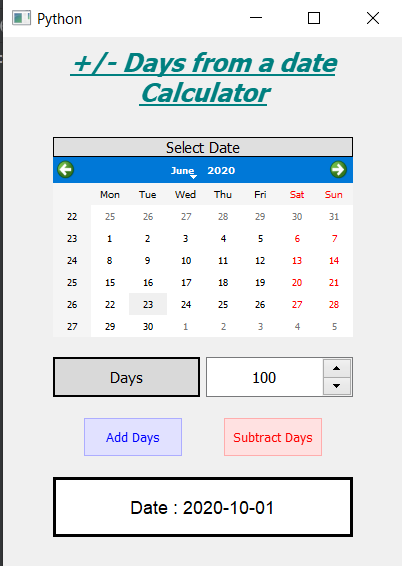# PyQt5 – Days From Date Calculator

• Last Updated : 24 Jun, 2020

In this article we will see how we can create a days from date calculator in PyQt5, days from date calculator is used to add or subtract the days from the selected date to get the new date. This calculator is used to get the exact date which would come after given number of days. Below is how the calculator will likePyQt5 is cross-platform GUI toolkit, a set of python bindings for Qt v5. One can develop an interactive desktop application with so much ease because of the tools and simplicity provided by this library. Below is the command to install the PyQt5

`pip install PyQt5`

GUI Implementation Steps :
1. Create a heading label that display the calculator name
2. Create label to show user to select the date
3. Create a QCalendarWidget object for user to select the date
4. Create a label and spin box for telling user to enter days and get the days
5. Create two push buttons for adding and subtracting the days
6. Create a label to show the calculated date

Back-End Implementation :
1. Add action to both the push button
2. Inside the add push button action get the days and call the calculate method and pass days as argument
3. Inside the subtract push button action get the days and make the days negative and call the calculate method and pass days as argument
4. Inside the calculate method get the selected date of the calendar
5. Add the passed days to the selected date
6. Show the new date with the help of result label

Below is the implementation

 `# importing libraries``from` `PyQt5.QtWidgets ``import` `*` `from` `PyQt5 ``import` `QtCore, QtGui``from` `PyQt5.QtGui ``import` `*` `from` `PyQt5.QtCore ``import` `*` `import` `datetime``import` `sys`` ` ` ` `class` `Window(QMainWindow):`` ` `    ``def` `__init__(``self``):``        ``super``().__init__()`` ` `        ``# setting title``        ``self``.setWindowTitle(``"Python "``)`` ` `        ``# width of window``        ``self``.w_width ``=` `400`` ` `        ``# height of window``        ``self``.w_height ``=` `530`` ` `        ``# setting geometry``        ``self``.setGeometry(``100``, ``100``, ``self``.w_width, ``self``.w_height)`` ` `        ``# calling method``        ``self``.UiComponents()`` ` `        ``# showing all the widgets``        ``self``.show()`` ` `    ``# method for components``    ``def` `UiComponents(``self``):``        ``# creating head label``        ``head ``=` `QLabel(``"+/- Days from a date Calculator"``, ``self``)`` ` `        ``head.setWordWrap(``True``)`` ` `        ``# setting geometry to the head``        ``head.setGeometry(``0``, ``10``, ``400``, ``60``)`` ` `        ``# font``        ``font ``=` `QFont(``'Times'``, ``15``)``        ``font.setBold(``True``)``        ``font.setItalic(``True``)``        ``font.setUnderline(``True``)`` ` `        ``# setting font to the head``        ``head.setFont(font)`` ` `        ``# setting alignment of the head``        ``head.setAlignment(Qt.AlignCenter)`` ` `        ``# setting color effect to the head``        ``color ``=` `QGraphicsColorizeEffect(``self``)``        ``color.setColor(Qt.darkCyan)``        ``head.setGraphicsEffect(color)`` ` `        ``# creating a label``        ``b_label ``=` `QLabel(``"Select Date"``, ``self``)`` ` `        ``# setting properties  label``        ``b_label.setAlignment(Qt.AlignCenter)``        ``b_label.setGeometry(``50``, ``100``, ``300``, ``20``)``        ``b_label.setStyleSheet(``"QLabel"``                              ``"{"``                              ``"border : 1px solid black;"``                              ``"background : rgba(70, 70, 70, 25);"``                              ``"}"``)``        ``b_label.setFont(QFont(``'Times'``, ``9``))`` ` `        ``# creating a calendar widget to select the date``        ``self``.calendar ``=` `QCalendarWidget(``self``)`` ` `        ``# setting geometry of the calendar``        ``self``.calendar.setGeometry(``50``, ``120``, ``300``, ``180``)`` ` `        ``# setting font to the calendar``        ``self``.calendar.setFont(QFont(``'Times'``, ``6``))`` ` ` ` `        ``# creating a label``        ``days_label ``=` `QLabel(``"Days"``, ``self``)`` ` `        ``# setting geometry to the label``        ``days_label.setGeometry(``50``, ``320``, ``147``, ``40``)`` ` `        ``# setting alignment``        ``days_label.setAlignment(Qt.AlignCenter)`` ` `        ``# setting stylesheet``        ``days_label.setStyleSheet(``"QLabel"``                                 ``"{"``                                 ``"border : 2px solid black;"``                                 ``"background : rgba(70, 70, 70, 35);"``                                 ``"}"``)`` ` `        ``days_label.setFont(QFont(``'Times'``, ``9``))`` ` `        ``# creating a spin box to get the days``        ``self``.days ``=` `QSpinBox(``self``)`` ` `        ``# setting geometry to the spin box``        ``self``.days.setGeometry(``203``, ``320``, ``147``, ``40``)`` ` `        ``# setting maximum value of spin box``        ``self``.days.setMaximum(``99999999``)`` ` `        ``# setting font and alignment``        ``self``.days.setFont(QFont(``'Times'``, ``9``))``        ``self``.days.setAlignment(Qt.AlignCenter)`` ` ` ` ` ` `        ``# creating a push button``        ``add ``=` `QPushButton(``"Add Days"``, ``self``)`` ` `        ``# setting geometry to the push button``        ``add.setGeometry(``80``, ``380``, ``100``, ``40``)`` ` `        ``# adding action to the button``        ``add.clicked.connect(``self``.add_action)`` ` `        ``# adding color effect to the push button``        ``color ``=` `QGraphicsColorizeEffect()``        ``color.setColor(Qt.blue)``        ``add.setGraphicsEffect(color)`` ` `        ``# creating a push button``        ``subtract ``=` `QPushButton(``"Subtract Days"``, ``self``)`` ` `        ``# setting geometry to the push button``        ``subtract.setGeometry(``220``, ``380``, ``100``, ``40``)`` ` `        ``# adding action to the  button``        ``subtract.clicked.connect(``self``.subtract_action)`` ` `        ``# adding color effect to the push button``        ``color ``=` `QGraphicsColorizeEffect()``        ``color.setColor(Qt.red)``        ``subtract.setGraphicsEffect(color)`` ` ` ` `        ``# creating a label to show result``        ``self``.result ``=` `QLabel(``self``)`` ` `        ``# setting properties to result label``        ``self``.result.setAlignment(Qt.AlignCenter)`` ` `        ``# setting geometry``        ``self``.result.setGeometry(``50``, ``440``, ``300``, ``60``)`` ` `        ``# making it multi line``        ``self``.result.setWordWrap(``True``)`` ` `        ``# setting stylesheet``        ``# adding border and background``        ``self``.result.setStyleSheet(``"QLabel"``                                  ``"{"``                                  ``"border : 3px solid black;"``                                  ``"background : white;"``                                  ``"}"``)`` ` `        ``# setting font``        ``self``.result.setFont(QFont(``'Arial'``, ``11``))`` ` `    ``# method called by the add push button``    ``def` `add_action(``self``):`` ` `        ``# get the days from the spin box``        ``days ``=` `self``.days.value()`` ` `        ``# call the calculate action``        ``self``.calculate(days)`` ` ` ` `    ``# method called by the subtract push button``    ``def` `subtract_action(``self``):`` ` `        ``# get the days from the spin box``        ``# make the days value negative``        ``days ``=` `0` `-` `self``.days.value()`` ` `        ``# call the calculate action``        ``self``.calculate(days)`` ` ` ` ` ` `    ``def` `calculate(``self``, days):`` ` `        ``# get the selected date of calendar``        ``selected_date ``=` `self``.calendar.selectedDate()`` ` `        ``# adding days to the selected days``        ``new_date ``=` `selected_date.addDays(days)`` ` `        ``# showing this date through label``        ``self``.result.setText(``"Date : "` `+` `new_date.toString(Qt.ISODate))`` ` `# create pyqt5 app``App ``=` `QApplication(sys.argv)`` ` `# create the instance of our Window``window ``=` `Window()`` ` `# start the app``sys.exit(App.``exec``())`

Output :

My Personal Notes arrow_drop_up